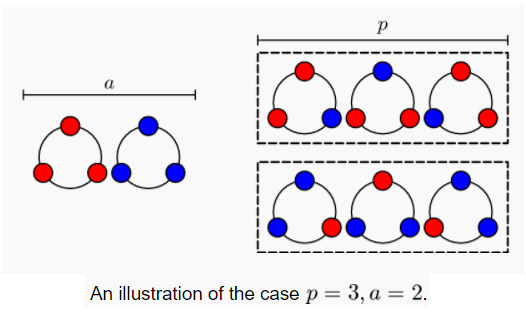## 费马🦓定理

· ☕ 1 分钟 · 👻 Victor
🏷️

## 费马小定理

$$a^{p} \equiv a \pmod p$$

$$a^{p-1} \equiv 1 \pmod p$$## 费马大定理

$$x^{n} + y^{n} = z^{n}$$Victor
Full Stack